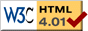# Inverted Double Pendulum

previous next
• Sim
• Graph
• Time Graph
• Multi Graph

Physics-based simulation of a vibrating double pendulum whose support point is oscillating rapidly up and down. Surprisingly, the inverted position where the pendulum stands straight up is stable.

Click near the pendulum to reposition it. The anchor point is also dragable. You can set gravity, frequency of oscillation, magnitude of oscillation, and damping (friction).

Experiments to try:

• Disturb one of the pendulums from its stable inverted position. Can it recover? How far can you disturb it?
• What if the masses are equal? Does that make the inverted position less stable?
• When the pendulums are in some random position, how hard is it to get them back to inverted?
• What is the slowest frequency that still has the stable inverted position?
• What is the range of amplitude that still has the stable inverted position?
• For a faster or slower frequency, does the range of amplitude change?
• Does the strength of gravity affect whether the inverted pendulum is stable?

The math for this simulation is similar to the Moveable Double Pendulum except that there is a periodic force for oscillating the anchor point. We assume the anchor/support point is so massive compared to the pendulum that it is not affected by the motion of the pendulums.

Also available are: open source code, documentation and a simple-compiled version which is more customizable.

Most of the references on the internet for a double inverted pendulum are for a different set up, where the double pendulum is attached to a moveable cart, similar to the cart + pendulum simulation. Also most of the these references are concerned with control theory for making an autonomous "brain" that can balance the inverted pendulum. In contrast, the experiment shown here uses only vertical vibration of the support point to make the inverted position stable.

previous next